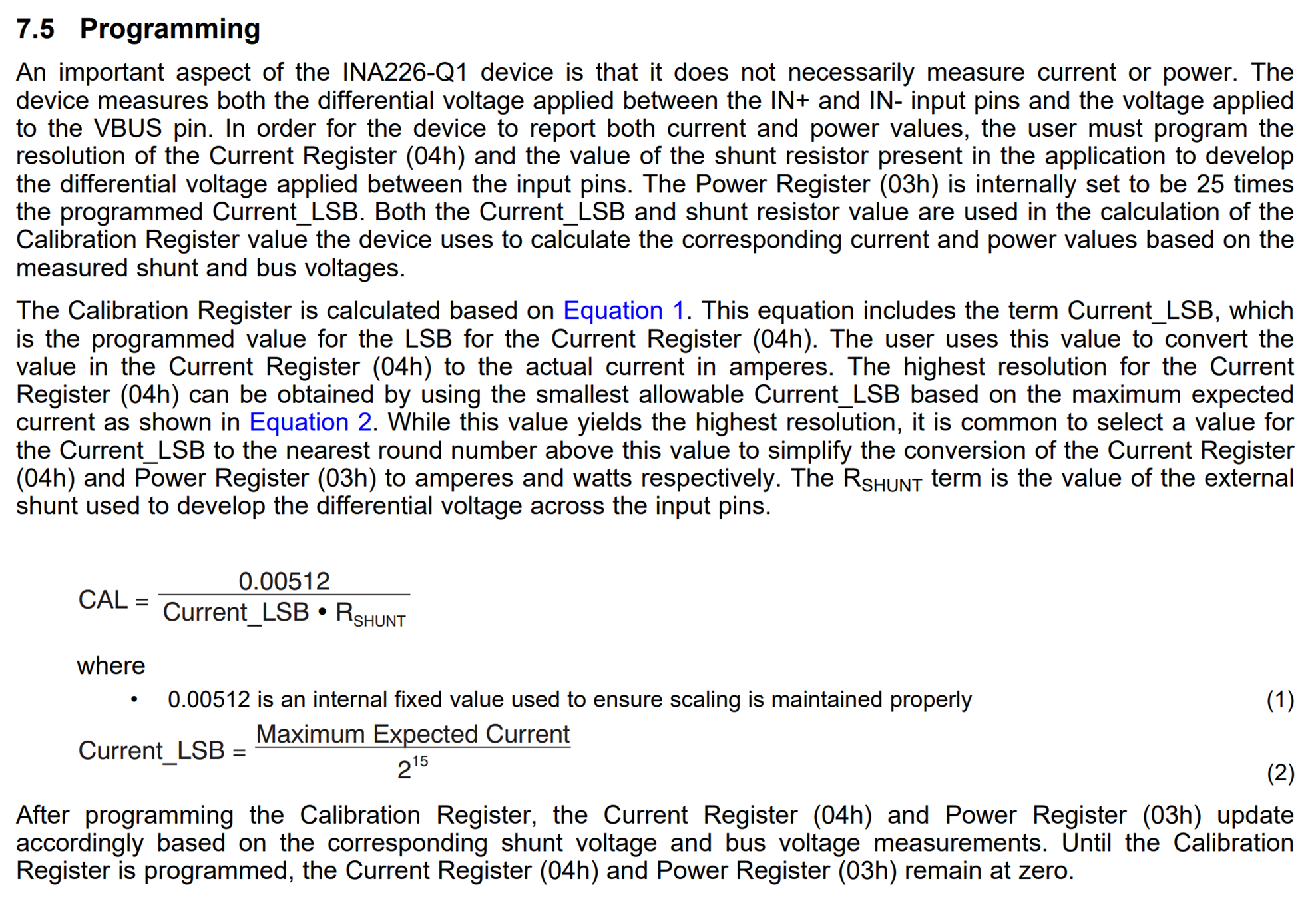If you have a related question, please click the "Ask a related question" button in the top right corner. The newly created question will be automatically linked to this question.

# INA226-Q1: How to convert actual current value?

Part Number: INA226-Q1

Hi team,

my customer would like to know if they read INA226-Q1 internal register value then they want to know how to calculate actual current or voltage value?

Ps. if we have convert excel tool will be preferred.

Thanks

• Hello Paul,

Here is how you convert the data for the shunt, bus, and current values:

Shunt Voltage: Multiply the register value by the shunt LSB value of 2.5µV

Bus Voltage: Multiply the register value by the bus LSB value of 1.25mV

Current: Multiply the register value by your chosen current LSB. See section 7.5 of the datasheet to see how to calculate your current LSB and Calibration register value. Here is a screenshot for convenience: